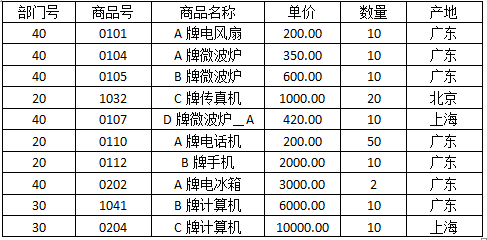#### 有以下计算公式若程序前面已在命令行中包含math.h文件，不能够正确计算上述公式的程序段是

• A.if(x>=0) y=sqrt(x); if(x<0) y=sqrt(-x);
• B.if(x>=0) y=sqrt(x); else y=sqrt(-x);
• C.y=sqrt(x); if(x<0) y=sqrt(-x);
• D.y=sqrt(x>=0?x :-x);2023年计算机等级考试题库